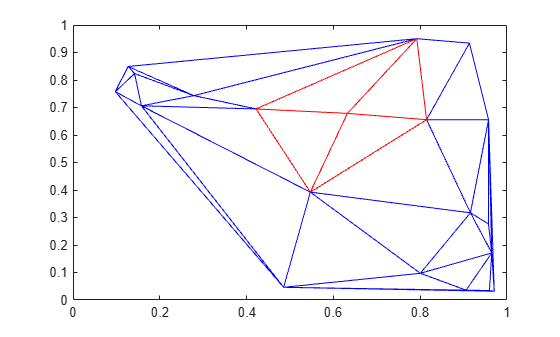# vertexAttachments

Triangles or tetrahedra attached to vertex

## Syntax

``V = vertexAttachments(TR)``
``V = vertexAttachments(TR,ID)``

## Description

````V = vertexAttachments(TR)` returns the IDs of the triangles or tetrahedra attached to every vertex in the triangulation `TR`. A triangle or tetrahedron ID is the row number of the corresponding triangle or tetrahedron in the property `TR.ConnectivityList`.The output `v` is an m-by-1 cell array where m is the number of vertices in `TR`. Each element of `V` contains the IDs of the attached triangles or tetrahedra corresponding to that vertex. The vertices can have a different number of attached triangles or tetrahedra depending on where they are located in the triangulation.```

example

````V = vertexAttachments(TR,ID)` returns the IDs of the triangles or tetrahedra attached to the vertices specified in `ID`. The vertex IDs in `ID` are the row numbers of the corresponding vertices in the property `TR.Points`.```

## Examples

collapse all

Locate and plot the attachments to a specified vertex in a 2-D Delaunay triangulation.

Create a Delaunay triangulation from a set of 2-D points.

```rng default; x = rand([20,1]); y = rand([20,1]); TR = delaunayTriangulation(x,y);```

Find the triangles attached to the 5th vertex.

```V = vertexAttachments(TR,5); V{:}```
```ans = 1×4 6 7 12 10 ```

Plot the triangulation, and plot the triangles attached to vertex 5 in red.

```triplot(TR) hold on triplot(TR(V{:},:),x,y,'Color','r')```## Input Arguments

collapse all

Triangulation representation, specified as a scalar `triangulation` or `delaunayTriangulation` object.

Data Types: `triangulation` | `delaunayTriangulation`

Vertex IDs of the query points, returned as a column vector. A vertex ID is the row number of the corresponding vertex in the `Points` property.

Data Types: `double`

## Version History

Introduced in R2013a